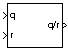# Quaternion Division

Divide quaternion by another quaternion

• Library:
• Aerospace Blockset / Utilities / Math Operations

•## Description

The Quaternion Division block divides a given quaternion by another. Aerospace Blockset™ uses quaternions that are defined using the scalar-first convention. The output is the resulting quaternion from the division or vector of resulting quaternions from division. For the quaternion forms used, see Algorithms.

## Ports

### Input

expand all

Dividend quaternions in the form of [q0, p0, ..., q1, p1, ... , q2, p2, ... , q3, p3, ...], specified as a quaternion or vector of quaternions.

Data Types: `double`

Divisor quaternions in the form of [s0, r0, ..., s1, r1, ... , s2, r2, ... , s3, r3, ...], specified as a quaternion or vector of quaternions.

Data Types: `double`

### Output

expand all

Output quaternion or vector of resulting quaternions from division.

Data Types: `double`

## Algorithms

The quaternions have the form of

`$q={q}_{0}+i{q}_{1}+j{q}_{2}+k{q}_{3}$`

and

`$r={r}_{0}+i{r}_{1}+j{r}_{2}+k{r}_{3}.$`

The resulting quaternion from the division has the form of

`$t=\frac{q}{r}={t}_{0}+i{t}_{1}+j{t}_{2}+k{t}_{3},$`

where

`$\begin{array}{l}{t}_{0}=\frac{\left({r}_{0}{q}_{0}+{r}_{1}{q}_{1}+{r}_{2}{q}_{2}+{r}_{3}{q}_{3}\right)}{{r}_{0}^{2}+{r}_{1}^{2}+{r}_{2}^{2}+{r}_{3}^{2}}\\ {t}_{1}=\frac{\left({r}_{0}{q}_{1}-{r}_{1}{q}_{0}-{r}_{2}{q}_{3}+{r}_{3}{q}_{2}\right)}{{r}_{0}^{2}+{r}_{1}^{2}+{r}_{2}^{2}+{r}_{3}^{2}}\\ {t}_{2}=\frac{\left({r}_{0}{q}_{2}+{r}_{1}{q}_{3}-{r}_{2}{q}_{0}-{r}_{3}{q}_{1}\right)}{{r}_{0}^{2}+{r}_{1}^{2}+{r}_{2}^{2}+{r}_{3}^{2}}\\ {t}_{3}=\frac{\left({r}_{0}{q}_{3}-{r}_{1}{q}_{2}+{r}_{2}{q}_{1}-{r}_{3}{q}_{0}\right)}{{r}_{0}^{2}+{r}_{1}^{2}+{r}_{2}^{2}+{r}_{3}^{2}}\end{array}$`

 Stevens, Brian L., Frank L. Lewis. Aircraft Control and Simulation, Second Edition. Hoboken, NJ: Wiley–Interscience.